## Breaking

CustomWritings.com - get an essay written for you by academic experts. Feel free to get writing assistance online.

## RD Sharma Solutions for Class 8 Chapter 15 Understanding Shapes- I (Polygons) Free Online

EXERCISE 15.1 PAGE NO: 15.5
1. Draw rough diagrams to illustrate the following:
(i) Open curve
(ii) Closed curve
Solution:
Here is the illustration of
(i) Open curve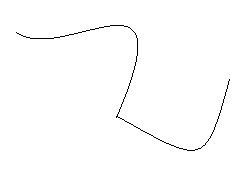(ii) Closed curve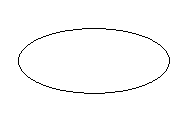2. Classify the following curves as open or closed: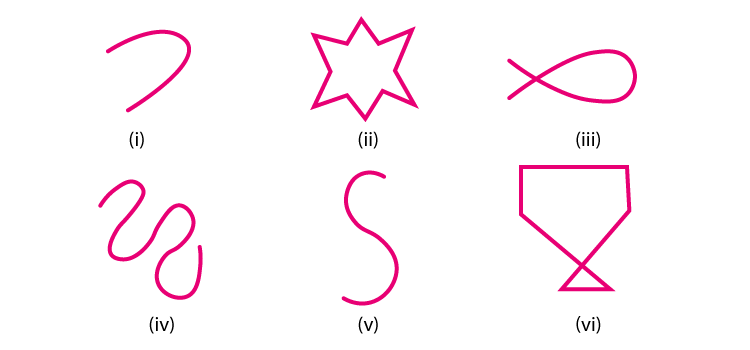Solution:
(i) Open curve
(ii) Closed curve
(iii) Closed curve
(iv) Open curve
(v) Open curve
(vi) Closed curve
3. Draw a polygon and shade its interior. Also draw its diagonals, if any.
Solution:
Here is the polygon with diagonals and with its interior shaded.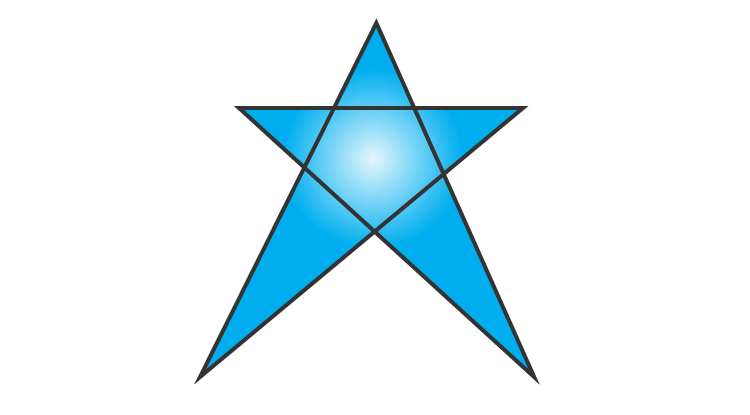4. Illustrate, if possible each one of the following with a rough diagram.
(i) A closed curve that is not a polygon.
(ii) An open curve made up entirely of line segments.
(iii) A polygon with two sides.
Solution:
(i) A closed curve that is not a polygon.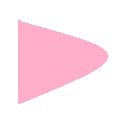(ii) An open curve made up entirely of line segments.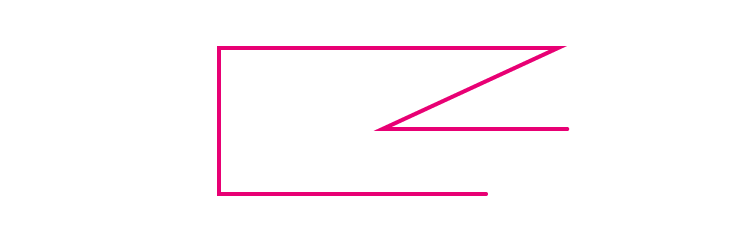(iii) A polygon with two sides.
A polygon with two sides is not possible because, a polygon should have minimum three sides.
5. Following are some figures: Classify each of these figures on the basis of the following:
(i) Simple curve (ii) Simple closed curve (iii) Polygon
(iv) Convex polygon (v) Concave polygon (vi) Not a curve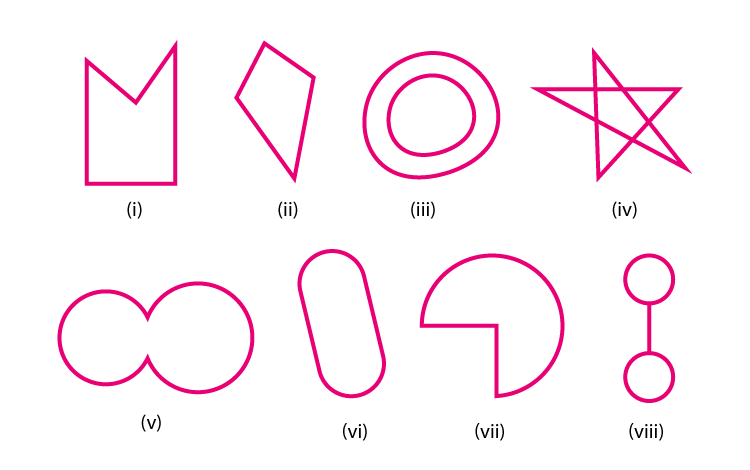Solution:
(i) It is a Simple Closed curve and a concave polygon. This is a simple closed curve and as a concave polygon all the vertices are not pointing outwards.
(ii) It is a Simple closed curve and a convex polygon. This is a simple closed curve and as a convex polygon all the vertices are pointing outwards.
(iii) It is Not a curve and hence it is not a polygon.
(iv) It is Not a curve and hence it is not a polygon.
(v) It is a Simple closed curve but not a polygon.
(vi) It is a Simple closed curve but not a polygon.
(vii) It is a Simple closed curve but not a polygon.
(viii) It is a Simple closed curve but not a polygon.
6. How many diagonals does each of the following have?
(i) A convex quadrilateral
(ii) A regular hexagon
(iii) A triangle
Solution:
(i) A convex quadrilateral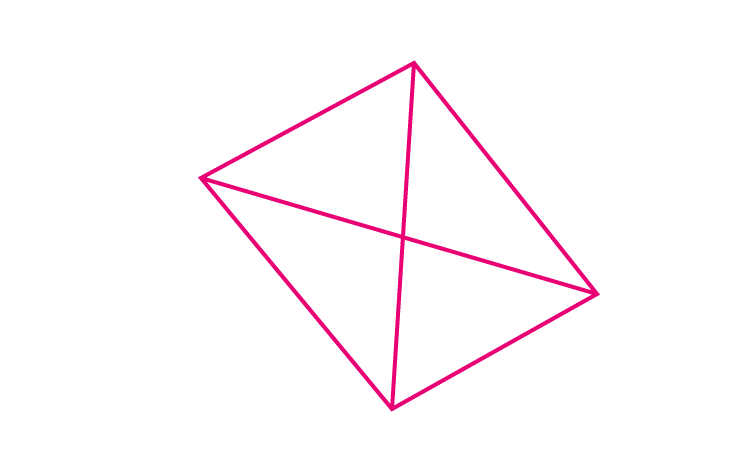For a convex quadrilateral we shall use the formula n(n-3)/2
So, number of diagonals = 4(4-3)/2 = 4/2 = 2
A convex quadrilateral has 2 diagonals.
(ii) A regular hexagon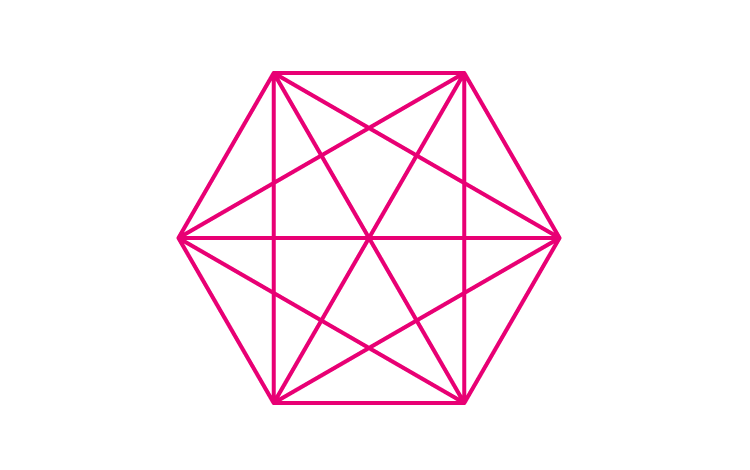For a regular hexagon we shall use the formula n(n-3)/2
So, number of diagonals = 6(6-3)/2 = 18/2 = 9
A regular hexagon has 9 diagonals.
(iii) A triangle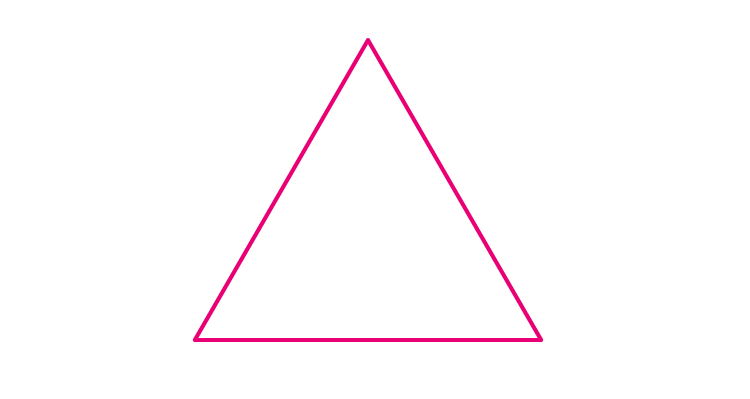For a triangle we shall use the formula n(n-3)/2
So, number of diagonals = 3(3-3)/2 = 0/2 = 0
A triangle has no diagonals.
7. What is a regular polygon? State the name of a regular polygon of
(i) 3 sides
(ii) 4 sides
(iii) 6 sides
Solution:
Regular Polygon: A regular polygon is an enclosed figure. In a regular polygon minimum sides are three.
(i) 3 sides
A regular polygon with 3 sides is known as Equilateral triangle.
(ii) 4 sides
A regular polygon with 4 sides is known as Rhombus.
(iii) 6 sides
A regular polygon with 6 sides is known as Regular hexagon.
Courtesy : CBSE